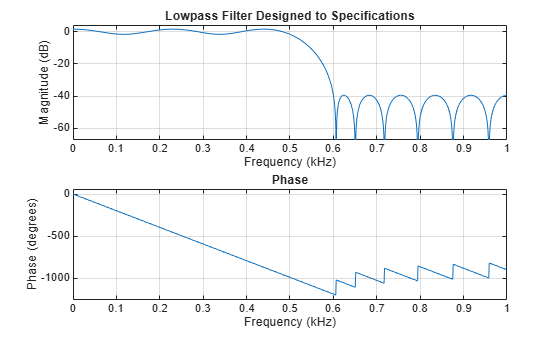Documentation

This is machine translation

Mouseover text to see original. Click the button below to return to the English version of the page.

firpmord

Parks-McClellan optimal FIR filter order estimation

Syntax

```[n,fo,ao,w] = firpmord(f,a,dev) [n,fo,ao,w] = firpmord(f,a,dev,fs) c = firpmord(f,a,dev,fs,'cell') ```

Description

`[n,fo,ao,w] = firpmord(f,a,dev)` finds the approximate order, normalized frequency band edges, frequency band amplitudes, and weights that meet input specifications `f`, `a`, and `dev`.

• `f` is a vector of frequency band edges (between 0 and Fs/2, where Fs is the sampling frequency), and `a` is a vector specifying the desired amplitude on the bands defined by `f`. The length of `f` is two less than twice the length of `a`. The desired function is piecewise constant.

• `dev` is a vector the same size as `a` that specifies the maximum allowable deviation or ripples between the frequency response and the desired amplitude of the output filter for each band.

Use `firpm` with the resulting order `n`, frequency vector `fo`, amplitude response vector `ao`, and weights `w` to design the filter `b` which approximately meets the specifications given by `firpmord` input parameters `f`, `a`, and `dev`.

```b = firpm(n,fo,ao,w) ```

`[n,fo,ao,w] = firpmord(f,a,dev,fs)` specifies a sampling frequency `fs`. `fs` defaults to 2 Hz, implying a Nyquist frequency of 1 Hz. You can therefore specify band edges scaled to a particular application's sampling frequency.

`c = firpmord(f,a,dev,fs,'cell')` generates a cell-array whose elements are the parameters to `firpm`.

Note

In some cases, `firpmord` underestimates or overestimates the order `n`. If the filter does not meet the specifications, try a higher order such as `n+1` or `n+2`.

Examples

collapse all

Design a minimum-order lowpass filter with a 500 Hz passband cutoff frequency and 600 Hz stopband cutoff frequency. Specify a sampling frequency of 2000 Hz. Require at least 40 dB of attenuation in the stopband and less than 3 dB of ripple in the passband.

```rp = 3; % Passband ripple rs = 40; % Stopband ripple fs = 2000; % Sampling frequency f = [500 600]; % Cutoff frequencies a = [1 0]; % Desired amplitudes```

Convert the deviations to linear units. Design the filter and visualize its magnitude and phase responses.

```dev = [(10^(rp/20)-1)/(10^(rp/20)+1) 10^(-rs/20)]; [n,fo,ao,w] = firpmord(f,a,dev,fs); b = firpm(n,fo,ao,w); freqz(b,1,1024,fs) title('Lowpass Filter Designed to Specifications')```Note that the filter falls slightly short of meeting the stopband attenuation and passband ripple specifications. Using `n+1` in the call to `firpm` instead of `n` achieves the desired amplitude characteristics.

Design a lowpass filter with a 1500 Hz passband cutoff frequency and 2000 Hz stopband cutoff frequency. Specify a sampling frequency of 8000 Hz. Require a maximum stopband amplitude of 0.1 and a maximum passband error (ripple) of 0.01.

```[n,fo,ao,w] = firpmord([1500 2000],[1 0],[0.01 0.1],8000); b = firpm(n,fo,ao,w);```

Obtain an equivalent result by having `firpmord` generate a cell array. Visualize the frequency response of the filter.

```c = firpmord([1500 2000],[1 0],[0.01 0.1],8000,'cell'); B = firpm(c{:}); freqz(B,1,1024,8000)```Algorithms

`firpmord` uses the algorithm suggested in . This method is inaccurate for band edges close to either 0 or the Nyquist frequency, `fs/2`.

References

 Rabiner, Lawrence R., and Otto Herrmann. “The Predictability of Certain Optimum Finite-Impulse-Response Digital Filters.” IEEE® Transactions on Circuit Theory. Vol.  20, Number 4, 1973, pp. 401–408.

 Rabiner, Lawrence R., and Bernard Gold. Theory and Application of Digital Signal Processing. Englewood Cliffs, NJ: Prentice-Hall, 1975, pp. 156–157.